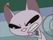cancel
Showing results for
Did you mean:Frequent Visitor

## Table.SelectRows Custom Column Looping Variable

I am new to Power Query.

I got this solution from another forum. I want to understand the logic behind this function.Funtion used = Table.AddColumn(Source, "Custom", each Table.SelectRows(Source,(x)=>x[Order ID]=[Order ID])) (highlighted part)

The above functions fell into the error when I tweaked it from this condition (x)=>x[Order ID]=[Order ID] to [Order ID]=[Order ID].

1 ACCEPTED SOLUTIONMemorable Member

Hi, @asif999 it's all about misleading "each" replacing (_) => and omitting _ later. Makes sense to use this in simple expressions but when you are dealing with iterator inside another iterator ... it's easy to get lost. Rewrite your step as below. Table.AddColumn iterates Source and (w) is a current row of that iteration. But w[Order ID] is another table you are iterating. (x) is a current item (row) of that iteration (over w[Order ID] table)

``Table.AddColumn(Source, "Custom", (w) => Table.SelectRows(Source,(x) => x[Order ID]= w[Order ID]))``

3 REPLIES 3Memorable Member

Hi, @asif999 it's all about misleading "each" replacing (_) => and omitting _ later. Makes sense to use this in simple expressions but when you are dealing with iterator inside another iterator ... it's easy to get lost. Rewrite your step as below. Table.AddColumn iterates Source and (w) is a current row of that iteration. But w[Order ID] is another table you are iterating. (x) is a current item (row) of that iteration (over w[Order ID] table)

``Table.AddColumn(Source, "Custom", (w) => Table.SelectRows(Source,(x) => x[Order ID]= w[Order ID]))``Memorable Member

@asif999 many thanks for accepting my answer as a solution... but I would like to reconsider my explanation.

Table.AddColumns still iterates Source table row by row. "w" is still a variable referring to the current row of iteration. But at any particular row of iteration "w" calls for another iteration function - Table.SelectRows. The last one asks for a table to iterate on - the original Source table -  and iteratates row by row with (x), selects those records of Source with [Order ID] = currently iterated row (w[Order ID]). It all looks like Table.Group by [Order ID] but it's not. It must be painfully slow.Frequent Visitor

Thanks for the explanation.

Now I got it 100%.

I was doubting the Table.SelectRows' iterator (x). And I was about to ask you the same.